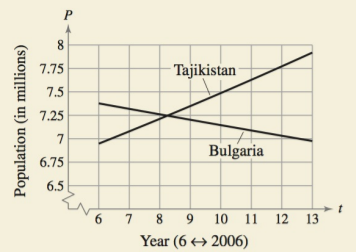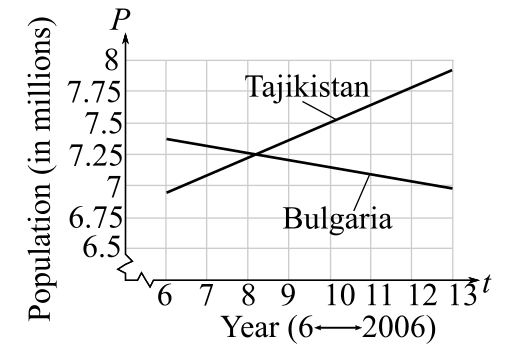Chapter 4.6, Problem 24E### Calculus: An Applied Approach (Min...

10th Edition
Ron Larson
ISBN: 9781305860919

#### Solutions

Chapter
Section### Calculus: An Applied Approach (Min...

10th Edition
Ron Larson
ISBN: 9781305860919
Textbook Problem
1 views

# HOW DO YOU SEE IT? The graph shows the populations (in millions) of Bulgaria and Tajikistan from 2006 through 2013 using exponential models. (Source: U.S. Census Bureau)(a) Determine whether the population of each country is modeled by exponential growth or exponential decay. Explain your reasoning.(b) Estimate the year when the two countries had the same population. What was this population?(a)

To determine

Whether the population of each country is modeled by exponential growth or decay from the following graph:Explanation

Given Information:

The provided graph is:

Consider the graph:

Exponential growth and decay:

If the rate of change of a positive quantity y with respect to time is proportional to the amount of quantity present at any time t, that is dydt=ky, then y is given by the equation, y=Ce

(b)

To determine

The year in which the population of both the country is same from the following graph:### Still sussing out bartleby?

Check out a sample textbook solution.

See a sample solution

#### The Solution to Your Study Problems

Bartleby provides explanations to thousands of textbook problems written by our experts, many with advanced degrees!

Get Started

#### Find more solutions based on key concepts